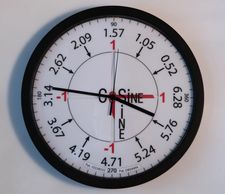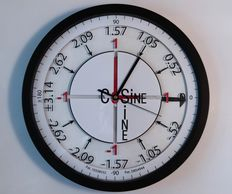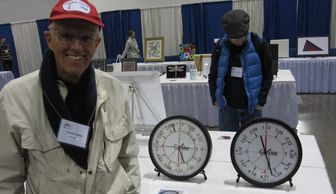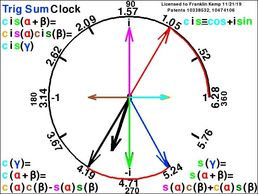#### (Co)Sine Clock Design 1 (CSC1)In this design (pats. 10338532, D854944) the hour hand is a moving angle indicator of radius length 1 from the clock center tracing the unit circle edge. Its location on the circle my be identified in two ways:  a Cartesian (x,y) coordinate pair and a polar point: the signed distance along the edge of the circle from the 3 o'clock position on the circle, positive if counterclockwise (ccw), negative if clockwise (cw).  The polar way uses only one number: signed arc length. Cosine and sine, proxies for x and y, respectively are parameterized by arc length. Their values are easy to estimate because they are the visible signed lengths of the red hands on the x and y axes (i.e. projections of the hour hand ) that run from 0 to -1 or 1. CSC1 runs through all (cosine, sine) pairs in twelve hours.  The hour hand becomes the diagonal of a rectangle if two sides parallel to the red hands are attached to its head. This rectangle splits into two congruent right triangles yielding the familiar right triangle definitions: hypotenuse (hour hand) , side adjacent (cosine), and side opposite (sine) of trigonometry where the angle is positive and less than a right angle.  Thus the unrestricted angle unit circle definitions of cosine and sine generalizes the simple right triangle of trigonometry affording the indispensable functions describing periodic phenomena. For example, the back and forth accelerated movement of the cosine hand reflects the simple harmonic motion of a pendulum along its arc while the up and down accelerated movement of the sine hand reflects the simple harmonic motion of a weight suspended by a spring.  Together their non-accelerated tangential movement reflects uniform circular motion.

#### (Co)Sine Clock Design 1 (CSC1)CSC2 is a novel  variant of CSC1 where the  minute hand is shortened becoming the angle indicator to the unit circle and the hour hand is lengthened, partly hidden under the circle, but extends outside the circle as the prominent arrow head.  CSC1 shows twelve numbers equally placed starting ccw from 2 o'clock with 0.52 and ending at 3 o'clock with 6.28. These numbers are the distances in fractions of the clock radius along the circle in place of the 12 hour numbers.  It is a geometrical fact the circumference C=2πr≈2(3.14)1=6.28 when divided by 12 yields ≈.52. But 12(0.52) = 6.24, so four increments must equal 0.52+0.01=0.53 to get 6.28. Where are they?  CSC2 runs through all (cosine, sine) pairs in one hour.  CSC2 shows  an alternative arc length labeling of CSC1 is available; namely, -3.14 to 3.14, ccw from the nine o'clock position. This labeling reveals that cosine and sine are even and odd functions of arc length, respectively.

#### (Co)Sine Clock Design 3 (CSC3)3004 Pataula Lane

Plano TX 75074-8765

(214)629-6031, (972)987-9574

President: Franklin Kemp, Ph D

cosineclock@gmail.com

Sales: Christopher Zhou

System Engineer: Christopher Zhou

cxqzhou88@gmail.com

(Co)Sine Clock Co was formed Oct. 1, 2018 in Plano, Texas to perfect, make, and market the world's only clock that tells time and trigonometry. The company puts a proprietary unit circle clock face (custom color) on a 14 inch atomic quartz clock. Since the unit circle defines cosines and sines not only of trigonometry but also more generally of all periodic phenomena, (Co)Sine Clock is a dynamic STEM educational tool, collector's item, and gift or award. (Co)Sine Clock comes with a one year warranty and certificate. CSC1 and CSC2 sell for \$100 and \$150, respectively,  plus tax and shipping. Computer generated clock designs CSC3 and TRIG SUM are executable programs licensed for \$25 each. To buy, please fill out the purchase form at the bottom of the page.

Videos of these clocks appear below.

#### (Co)Sine Clock Design 3 (CSC3)This software (Co)Sine Clock design (pats. 10338532, 10474106) is for license. Here the red arrow second hand is the angle indicator. Its projection on the x axis is the cosine (orange  arrow .707) and on the y axis is the sine (magenta arrow -.707).  Tangent and cotangent values are arrows on the sides of the enclosing unit square where the second hand would hit the square if extended. The display shows shows a magenta tangent arrow -1.000.  The cotangent arrow, its reciprocal, is not shown since the software only plots one arrow hitting the square. Tangent (cotangent) arrows are bigger sine (cosine) arrows. Toggling the lime or orange box switches the angular labeling between 0 to 2π and -π to π.  Clicking elsewhere stops or starts time. The short blue line segment represents angular velocity Δθ/Δt=ω=-6.28 radians/60sec ≈ -.1 radians/sec and indicates uniform circular motion.  The rate of change of cosine (sine) with respect to θ on the unit circle is the limit of the run (rise) of the tangent (the blue line segment Δθ) to the unit circle at the point. Its slope is the negative reciprocal of tan(θ)=s/c; i.e -c/s. For a change of Δθ>0 along the tangent line, the run is -sΔθ and the rise is cΔθ. But the run (rise) is the change in the cosine (sine); so, c'=-s and s'=c, upon dividing out Δθ. From this it follows that c''+c=0 and s''+s=0 because c''=(c')'=(-s)'=-c and s''=(s')'=(c)'=-s or another right angle ccw rotation of the unit right triangle yields the negative reciprocal -s/-c  opposing the arrow of the initial unit right triangle. The two equations describe simple harmonic motion in the x and y directions, respectively. Seeing numerical values and the movement of the point on the unit circle support the mathematics. For example, multiplying  the numerical values c'(s') and c''(s'') by Δθ=-.001 show how c(s) and c'(s') increase or decrease. Another example: since apparently since s'(0)=1=cos(0) and s'(π/2)=0=c(π/2) and likewise c'(0)=0=s(0) and c'(π/2)=-1=-s(π/2) promote the formulas for the derivatives of s and c and their bounds being between -1 and 1.

#### Trig Sum DerviationThis design (pats. 10338532, 10474106) tells time and shows the  "sum" of  the red second hand α and the black minute hand β is the blue hand γ. Including cosine and sine hands, there are ten hands altogether. This design has an imaginary y-axis so the hands represent complex numbers. cis is short for cos+i sin (i = √ -1). The blue hand is the complex product of the red second hand times the black minute hand which manifests the trigonometric sum identities for cosine and sine displayed on the clock face in both complex and real form. The complex multiplication shows that the product of any two unit hands is located at the angle that is the sum of the angles of the two factors. This is a logarithmic property: multiplication is reduced to summation. This observation leads to DeMoivre's roots of unity. DeMoivre's Theorem is the same as producing a unit hand when multiplied by itself a number of times produces the unit hand at (1,0).  For example, the unit hand at (-1,0) when multiplied by itself will land at (1,0),  a hand at 2π/3 or 4π/3 when multiplied by themselves twice more are roots of unity, etc.

#### Trig Sum DerviationPeter Lax (Linear Algebra, 2nd ed. p. 78) observes that the squared distances of vectors x and y in the identity  Σ(x-y)² = Σx² - 2Σxy + Σy² are invariant in any Cartesian coordinate system; thus  the dot product  Σxy must be also. He puts the tails of x and y  together and chooses a special coordinate system where the first coordinate axis runs along x and the second axis is in the plane spanned by x and y. In this system x = (√Σx²,0,..,0) and y = (√Σy²cosθ, √Σy²sinθ,...) so the dot product Σxy = √Σx²√Σy²cosθ is the length of x times the projection of y on the axis containing x and θ is the angle between x and y.

c(α+β) is the projection of the unit arrow to angle α+β on the unit arrow to (1,0). This projection is invariant under rotation so rotating cw by α we arrive at the projection of arrow to β = (c(β),s(β)) on arrow -α = (c(-α),s(-α)); hence, by Lax c(α+β) is the inner product of arrow β on arrow -α giving c(-α)c(β)+s(-α)s(β) = c(α)c(β)-s(α)s(β) and s(α+β) = c(π/2-(α+β)) = c(π/2-α)c(-β)-s(π/2-α)s(-β) = s(α)c(β)+c(α)s(β).  All the arrows are unit vectors, but the unit arrow to α+β is not the sum of arrow α and arrow β.  It is more akin to a product of arrow α and arrow β in order to be a unit arrow.  Indeed, arrow α and arrow β correspond to cis(α) and cis(β) interpreted as vectors. To check that c(α+β) is the dot product of  arrow -α and arrow β, first, freeze the Trig Sum display (i.e. touch screen or key pad or click mouse), second, imagine a flip of the red second hand (arrow α) across the x axis yielding arrow -α and, finally project it on the black minute hand (arrow β) to get the cosine of the blue hand (arrow α+β) i.e the cyan arrow.

## USE OF COSINE AND SINE"PIE ARE SQUARE" by M. Hewitt.  What and where is the colored digit of π appearing once?

Trigonometry solves any triangle for angles and lengths by decomposing it into two right triangles.  Every right triangle is similar to a "unit" right triangle with a particular (cosine, sine) pair: cosine²+sine²=1² whose pairs are taken as known for every point on the unit circle thereby enabling the trigonometric laws of cosines, sines, and tangents.  CSC3 will freeze a pair by touching the screen, keypad, or left clicking a mouse. Use Esc to exit CSC3.

Physics: Superposition: The radial hand to the unit circle can be interpreted as a force (vector) of strength 1. It is equivalent to the addition of two axial forces (vectors), its cosine and sine. A pull in the direction of radial position is the net pull of its cosine and sine along their axes. Engineers add forces by decomposing them into their axial strengths.

Periodic Motion: simple harmonic motion, pendulums, springs, waves (e.g. light, sound, music, alternating current), orbits, rotations.